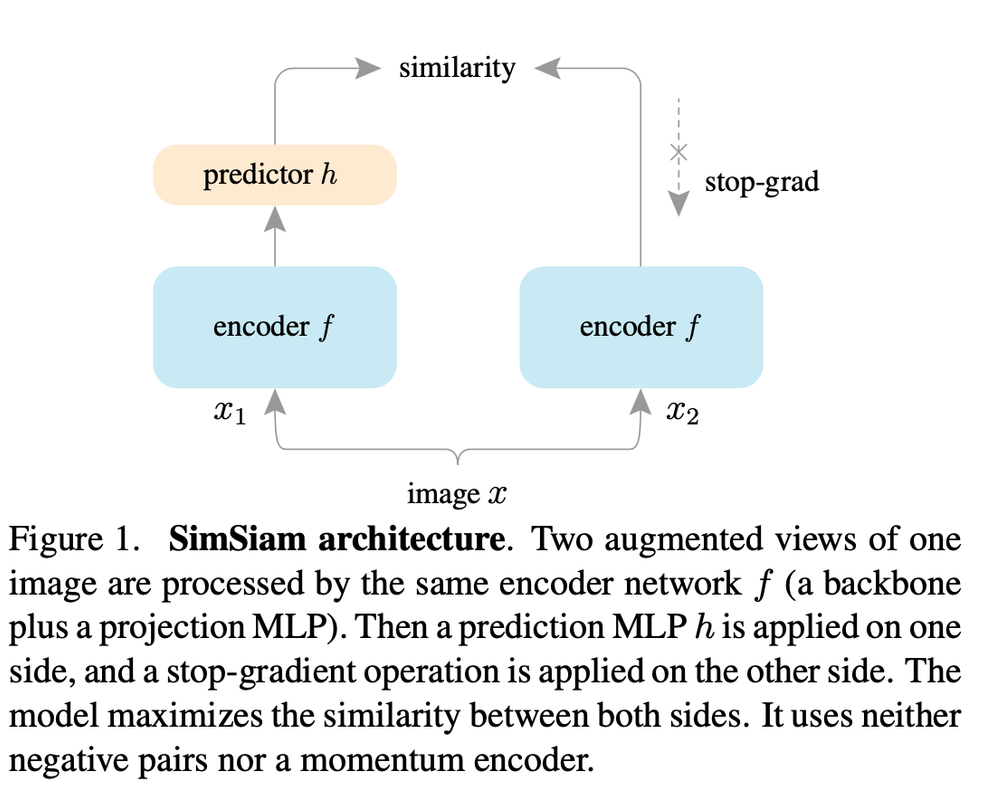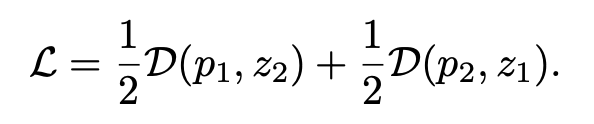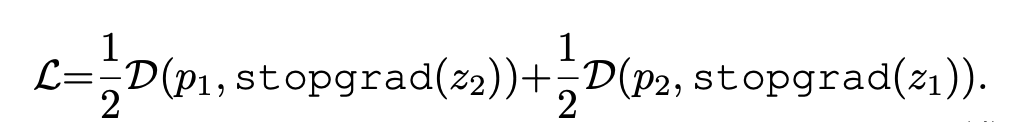# Exploring Siamese Network

Exploring Simple Siamese Representation Learning

• Siamese network is two parallel network sharing same weights to maximize the similarity between each network input.
• Siamese network is trained in an unsupervised manner. A image is augmented to create a positive pair for which the similarity score is ideally 1, on the other hand, a negative pair will have a similarity score of 0.
• Siamese network face collapsing problem i.e when all outputs collapsing to a constant.
• To avoid collapsing different strategies such as contrastive learning, clustering are tried. In contrastive learning, the negative pairs precludes the constant output. More variety in negative pair, forces the network to vary the output. The idea is, constant output occurs when there is negative and positive pair repeatablity, forcing the model to fixate on its output, by introducing more variations between the pair might help.
• In clustering, they alternate between clustering the representations and learning to predict the cluster assignment.
• SimSiam proves to prevent collapsing by using stop-gradient operation.

### Method• The architecture takes two input of randomly augmented views(x1 and x2) from an image (x).

• The encoded representation of f(x1) is fed to a MLP, the output is h(f(x1)) .

• MLP has batch normalisation to each fc layer. The output has no RELU, the hidden fc is 2048 dimension.

• Two encoder shares weights.

• Minimization of negative cosine similarity of two output vectors p1 = h(f(x1)) and z2 = f(x2) is the training objective.

• The symmetrized loss is given by